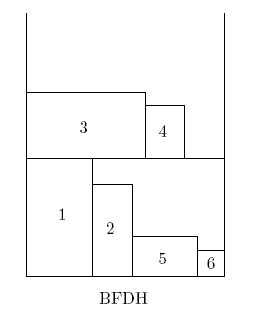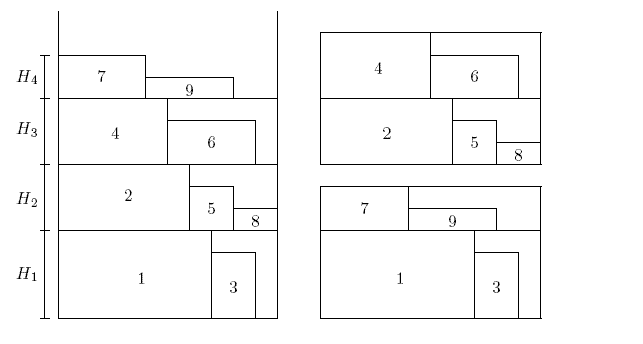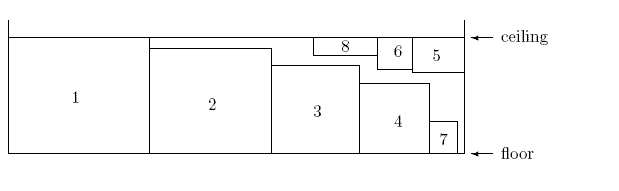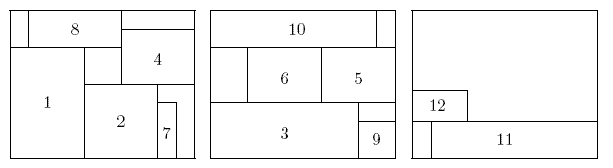# Survey on two-dimensional packing

 1.  Strip packing 2.  Bin packing 2.1  Two-phase algorithms 2.2  One-phase

# 1 Strip packing

In the two-dimensional strip packing problem, we are given a strip of a finite width W but infinite height, and a set of rectangular items each of width at most W. The objective is to pack all the items into the strip to minimize the height used. The items may neither overlap nor be rotated. We describe here a list of efficient (off-line) packing algorithms. A common approach is level-oriented, the items are packed from left to right, in rows forming levels. Within the same level, all items are packed so that their bottoms align. The first level is the bottom of the strip and subsequent levels are defined by the height of the tallest item on the previous level. Some algorithms start by sorting the items by non-increasing height, they are usually named Decreasing Height (DH). The first three DH algorithms reviewed below are level-oriented. Given an approximation algorithm A, let A(I) and OPT(I) denote the height used by A and the optimal algorithm, respectively, for an instance I. The asymptotic bounds stated below assume that the width of the strip and the items is normalized so that the strip is of width 1.

1. First-Fit Decreasing Height (FFDH) algorithm
FFDH packs the next item R (in non-increasing height) on the first level where R fits. If no level can accommodate R, a new level is created.
Time complexity of FFDH: O(n·log n).
Approximation ratio: FFDH(I)<=(17/10)·OPT(I)+1; the asymptotic bound of 17/10 is tight.2. Next-Fit Decreasing Height (NFDH) algorithm
NFDH packs the next item R (in non-increasing height) on the current level if R fits. Otherwise, the current level is "closed" and a new level is created.
Time complexity: O(n·log n).
Approximation ratio: NFDH(I) <= 2·OPT(I)+1; the asymptotic bound of 2 is tight.3. Best-Fit Decreasing Height (BFDH) algorithm
BFDH packs the next item R (in non-increasing height) on the level, among those that can accommodate R, for which the residual horizontal space is the minimum. If no level can accommodate R, a new level is created.4. Bottom-Left (BL) Algorithm
BL first order items by non-increasing width. BL packs the next item as near to the bottom as it will fit and then as close to the left as it can go without overlapping with any packed item. Note that BL is not a level-oriented packing algorithm.
Time complexity: O(n2 ).
Approximation ratio: BL(I)<=3·OPT(I) .
5. Baker's Up-Down (UD) algorithm 
UD uses a combination of BL and a generalization of NFDH. The width of the strip and the items are normalized so that the strip is of unit width. UD orders the items in non-increasing width and then divides the items into five groups, each with width in the range (1/2, 1], (1/3,1/2], (1/4,1/3], (1/5,1/4], (0,1/5]. The strip is also divided into five regions R1, ··· , R5. Basically, some items of width in the range (1/i+1, 1/i], for 1 <= i <= 4, are packed to region Ri by BL. Since BL leaves a space of increasing width from top to bottom at the right side of the strip, UD takes this advantage by first packing the item to Rj for j = 1, ··· , 4 (in order) from top to bottom. If there is no such space, the item is packed to Ri by BL. Finally, items of size at most 1/5 are packed to the spaces in R1, ··· , R4 by the (generalized) NFDH algorithm. Again if there is no space in these regions, the item is packed to R5 using NFDH.
Approximation ratio: UD(I) <= (5/4) · OPT(I)+(53/8)H, where H is the maximum height of the items; the asymptotic bound of 5/4 is tight .
6. Reverse-fit (RF) algorithm 
RF also normalizes the width of the strip and the items so that the strip is of unit width. RF first stacks all items of width greater than 1/2. Remaining items are sorted in non-increasing height and will be packed above the height H0 reached by those greater than 1/2. Then RF repeats the following process. Roughly speaking, RF packs items from left to right with their bottom along the line of height H0 until there is no more room. Then packs items from right to left and from top to bottom (called reverse-level) until the total width is at least 1/2. Then the reverse-level is dropped down until (at least) one of them touches some item below. The drop down is somehow repeated and we refer the reader to  for more details.
Approximation ratio: RF(I) <= 2·OPT(I) .
7. Steinberg's algorithm 
Steinberg's algorithm, denoted as M in the paper, estimates an upper bound of the height H required to pack all the items such that it is proved that the input items can be packed into a rectangle of width W and height H. They then define seven procedures (with seven conditions), each to divide a problem into two smaller ones and solve them recursively. It has been showed that any tractable problem satisfies one of the seven conditions.
Approximation ratio: M(I) <= 2·OPT(I).
8. Split-Fit algorithm (SF)  
SF divides items into two groups, L1 with width greater than 1/2 and L2 at most 1/2. All items of L1 are first packed by FFDH. Then they are arranged so that all items with width more than 2/3 are below those with width at most 2/3. This creates a rectangle R of space with width 1/3. Remaining items in L2 are then packed to R and the space above those packed with L1 using FFDH. The levels created in R are considered to be below those created above the packing of L1.
Approximation ratio: SF(I) <= (3/2) ·OPT(I) + 2; the asymptotic bound of 3/2 is tight .
9. Sleator's algorithm  
Sleater's algorithm consists of four steps: (1) all items of width greater than 1/2 are packed on top of one another in the bottom of the strip. Suppose h0 is the height of the resulting packing All subsequent packing will occur above h0. (2) Remaining items are ordered by non-increasing height. A level of items are packed (in non-increasing height order) from left to right along the line of height h0. (3) A vertical line is then drawn in the middle to cut the strip into two equal halves (note this line may cut an item that is packed partially in the right half). Draw two horizontal line segments of length one half, one across the left half (called the left baseline) and one across the right half (called the right baseline) as low as possible such that the two lines do not cross any item. (4) Choose the left or right baseline which is of a lower height and pack a level of items into the corresponding half of the strip until the next item is too wide. A new baseline is formed and Step (4) is repeated on the lower baseline until all items are packed.
Time complexity: O(n ·log n).
The approximation ratio of Sleator's algorithm is 2.5 which is tight .

# 2 Bin packing

In the two-dimensional bin packing problem, we are given an unlimited number of finite identical rectangular bins, each having width W and height H, and a set of n rectangular items with width wj <= W and height hj, for 1 <= j <= n. The problem is to pack, without overlap, all the items into the minimum number of bins. The items cannot be rotated. Most of the off-line algorithm in the literature are of greedy type, and can be classified into two families:
• one phase algorithms directly pack the items into the finite bins;
• two phase algorithms start by packing the items into a single strip, i.e., a bin having width W and infinite height. In the second phase, the strip solution is used to construct a packing into finite bins.

## 2.1 Two-phase algorithms

The following two phase algorithms make use of some level-oriented algorithms to obtain a strip packing. Suppose H1, H2, ··· are the heights of the resulting levels of the strip packing. A finite bin packing solution is then obtained by solving a one-dimensional bin packing problem (with item size Hi and bin capacity H).
1. Hybrid First-Fit (HFF) 
In the first phase, a strip packing is obtained by the FFDH algorithm. The second phase adopts the First-Fit Decreasing (FFD) algorithm, which packs an item to the first bin that it fits or start a new bin otherwise.
Time complexity: O(n·log n).
The approximation ratio of HFF is 17/8 . The bound is not proved to be tight: the best lower bound of HFF known is 91/45.2. Hybrid Next-Fit (HNF) 
NFDH is adopted in the first phase. In the second phase, the one-dimensional bin packing problem is solved by the Next-Fit Decreasing (NFD) algorithm, which packs an item to the current bin if it fits, or start a new bin otherwise.
Time complexity: O(n·log n).
The approximation ratio of HNF is 3.382 .
3. Hybrid Best-Fit (HBF) 
In the first phase, BFDH strategy is adopted. The second phase adopts the Best-Fit Decreasing (BFD) algorithm, which packs an item to the best bin (one with the smallest space left) that it fits or start a new bin otherwise.
4. Floor-Ceiling (FC) algorithm  
Consider a particular level, the horizontal line defined by the top (resp. bottom) edge of the tallest item is called the ceiling (resp. floor) of the level. In the first phase, FC packs an item into a level either from left to right with their bottom edge on the level floor or from right to left, with their top edge on the level ceiling. The first item packed on a ceiling must be one which cannot be packed on the floor in the same level. The order of preference when FC packs an item in the first phase: (i) on a ceiling (provided that the requirement above is satisfied), using best-fit (BF) algorithm; (ii) on a floor, using BF algorithm; (iii) on the floor of a new level.
In the second phase, the levels are packed into finite bins, either by BFD or by an exact algorithm for the one-dimensional bin packing problem, halted after a prefixed number of iterations.
Time complexity: The implementation of the first phase given in  requires O(n3 ) time, while the complexity of the second one depends on the selected algorithm.## 2.2 One-phase

1. Finite Next-Fit (FNF) 
FNF directly packs the items into finite bins in the same way as HNF.
Time complexity: O(n·log n).
2. Finite First-Fit (FFF) 
FFF adopts instead the FFDH strategy. An item is packed on the lowest level of the first bin where it fits; if no level can accommodate it, a new level is created in the first bin having sufficient vertical space, otherwise, the new level is created in a new bin.
Time complexity: O(n·log n).3. Finite Bottom-left (FBL) 
FBL does not pack the items by levels. Berkey and Wang  proposed the BL approach for the finite bin case. Their Finite Bottom-Left (FBL) algorithm initially sorts the items by non-increasing width. The next item is packed in the lowest position of any existing bin, left justified; if no bin can allocate it, a new one is started.
Time complexity: Both Chazelle  and Berkey and Wang  have provided an O(n2 ) implementation of the algorithm.
4. Next Bottom-left (NBL) 
NBL is similar to FBL except that at any time only one bin is opened for packing. Once an item cannot be packed a bin, the bin is closed and will not be used for further packing.